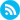RSS tips

#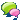Discussion forum

##CAD discussionCAD discussion forum - ask any CAD-related questions here, share your CAD knowledge on AutoCAD, Inventor, Revit and other Autodesk software with your peers from all over the world. To start a new topic, choose an appropriate forum.

Please abide by the rules of this forum.

How to post questions: register or login, go to the specific forum and click the NEW TOPIC button.Forum Home > EN > AutoCADLatest Posts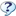FAQ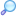Search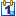EventsRegisterLogin

# How find a drawing with a specific layer name?

AuthorTopic SearchTopic Options
remcokoedootGroupie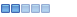Joined: 16.Oct.2013
Location: Netherlands
Status: Offline
Points: 33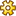Post OptionsThanks(0)Quote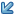ReplyTopic: How find a drawing with a specific layer name?
Posted: 11.Nov.2022 at 20:34
I'm looking for a way through a lisp routine to search various folders drawings (.dwg files) for a specific layer name.
The result in an excel list of the found dwg files where the specific layer is located.

Edited by remcokoedoot - 11.Nov.2022 at 20:35
With kind regards,

Remco Koedoot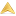remcokoedootGroupieJoined: 16.Oct.2013
Location: Netherlands
Status: Offline
Points: 33Post OptionsThanks(1)QuoteReplyPosted: 13.Nov.2022 at 12:08
I have a lisp routine that will scan in a folder of drawings for all layer names. It must be in a atnother solution. To specify to search various folders drawings (.dwg files) for a specific layer name. The result in an excel list of the found dwg files where the specific layer is located.

(defun c:CheckLayers ( / *error* DBX DOCLST FILES FLAG LAYER_LIST ODOC OFILE OUTFILE SHELL )

(defun *error* (msg)
(ObjRelease (list Shell dbx))
(and ofile (= (type ofile) 'FILE) (close ofile))

(or (wcmatch (strcase msg) "*BREAK,*CANCEL*,*EXIT*")
(princ (strcat "\n** Error: " msg " **")))
(princ)
)

*doc  (cond (*doc ) ((vla-get-ActiveDocument *acad))))

(if (and (setq Files   (GetAllFiles nil t "*.dwg"))
(setq outfile (getfiled "Output File" "" "csv" 1)))
(progn

(setq DocLst
(cons
(cons (strcase (vla-get-FullName doc)) doc) DocLst
)
)
)

(setq dbx (ObjectDBXDocument))

(foreach dwg Files

(cond
(  (setq flag
(and
(setq oDoc
(cdr (assoc (strcase dwg) DocLst))
)
)
)
)
(t
(setq flag
(not
(vl-catch-all-error-p
(vl-catch-all-apply
(function vla-open) (list dbx dwg)
)
)
)
)
(setq oDoc dbx)
)
)

(setq Layer_List
(if flag
(cons (cons dwg (GetLayerProperties oDoc)) Layer_List)
(cons (cons dwg '(("**Error Opening this Drawing **"))) Layer_List)
)
)
)

(princ (strcat "\n<< " (itoa (length Files)) " Drawings Processed >>"))
)
(princ "*Cancel*")
)

(vlax-release-object dbx) (gc) (gc)

(if (and Layer_List (setq ofile (open outfile "w")))
(progn
(mapcar
(function
(lambda (x)
(write-line (car x) ofile)
(write-line (MakeString '("Name" "Colour" "LineType" "LineWeight") (chr 32)) ofile)
(mapcar
(function
(lambda (y)
(write-line
(MakeString y (chr 32)) ofile
)
)
)
(cdr x)
)
(write-line "\n" ofile)
)
)
Layer_List
)
(close ofile)
)
(princ "\n*Cancel*")
)
(princ)
)

(defun ObjectDBXDocument ( / acVer )

(if (< (setq acVer (atoi (getvar "ACADVER"))) 16) "ObjectDBX.AxDbDocument"
(strcat "ObjectDBX.AxDbDocument." (itoa acVer))
)
)
)

(defun GetAllFiles ( Dir Subs Filetype / GetSubFolders Shell Fold Dir )

(defun GetSubFolders ( folder / _f )
(mapcar
(function
(lambda ( f ) (setq _f (strcat folder "\\" f))
(cons _f (apply (function append)
(GetSubFolders _f)))
)
)
(cddr (vl-directory-files folder nil -1))
)
)

(cond
( (not
(or
(and Dir (vl-file-directory-p Dir))
(progn
(setq Shell (vla-getInterfaceObject
Fold  (vlax-invoke-method Shell 'BrowseForFolder
(vlax-release-object Shell)

(if Fold
(progn
(setq Dir (vlax-get-property
(vlax-get-property Fold 'Self) 'Path))
(vlax-release-object Fold)

(and (= "\\" (substr Dir (strlen Dir)))
(setq Dir (substr Dir 1 (1- (strlen Dir)))))

Dir
)
)
)
)
)
)
( (apply (function append)
(vl-remove (quote nil)
(mapcar
(function
(lambda (Filepath)
(mapcar
(function
(lambda (Filename)
(strcat Filepath "\\" Filename)
)
)
(vl-directory-files Filepath Filetype 1)
)
)
)
(append (list Dir)
(apply (function append)
(if subs (GetSubFolders Dir))
)
)
)
)
)
)
)
)

(defun GetLayerProperties ( doc / lst )
(vlax-for lay (vla-get-Layers doc)
(setq lst
(cons
(mapcar
(function
(lambda ( property )
(vl-princ-to-string
(vlax-get-property lay property)
)
)
)
'(Name Color Linetype LineWeight)
)
lst
)
)
)
(vl-sort lst
(function
(lambda (a b) (< (car a) (car b)))
)
)
)

(defun MakeString  ( lst del / Pad str x i )
(setq i 10)

(defun Pad ( Str Del Len )
(while (>= (strlen Str) Len) (setq Len (+ Len 5)))
(while (< (strlen Str) Len)
(setq Str (strcat Str Del))
)
Str
)

(apply (function strcat)
(reverse
(cons (last lst)
(mapcar
(function
(lambda ( \$str )
(Pad \$str del (setq i (abs (- 40 i))))
)
)
(cdr (reverse lst))
)
)
)
)
)

Edited by remcokoedoot - 13.Nov.2022 at 12:11
With kind regards,

Remco KoedootremcokoedootGroupieJoined: 16.Oct.2013
Location: Netherlands
Status: Offline
Points: 33Post OptionsThanks(0)QuoteReplyPosted: 17.Nov.2022 at 09:52remcokoedoot wrote:I have this lisp routine that will scan in a folder of drawings for all layer names. It must be in a atnother solution. To specify to search various folders drawings (.dwg files) for a specific layer name. The result in an excel list of the found dwg files where the specific layer is located.  By running the lisp, how to wtite the input (layer name) in a shell-window and not by the routine thats results all layer names?(defun c:CheckLayers ( / *error* DBX DOCLST FILES FLAG LAYER_LIST ODOC OFILE OUTFILE SHELL ) (vl-load-com) (defun *error* (msg)   (ObjRelease (list Shell dbx))   (and ofile (= (type ofile) 'FILE) (close ofile))      (or (wcmatch (strcase msg) "*BREAK,*CANCEL*,*EXIT*")       (princ (strcat "\n** Error: " msg " **")))   (princ) ) (setq *acad (cond (*acad) ((vlax-get-acad-object)))       *doc  (cond (*doc ) ((vla-get-ActiveDocument *acad)))) (if (and (setq Files   (GetAllFiles nil t "*.dwg"))          (setq outfile (getfiled "Output File" "" "csv" 1)))   (progn          (vlax-for doc (vla-get-Documents *acad)       (setq DocLst         (cons           (cons (strcase (vla-get-FullName doc)) doc) DocLst         )       )     )           (setq dbx (ObjectDBXDocument))          (foreach dwg Files       (cond         (  (setq flag              (and                (setq oDoc                  (cdr (assoc (strcase dwg) DocLst))                )              )            )          )         (t           (setq flag             (not               (vl-catch-all-error-p                 (vl-catch-all-apply                   (function vla-open) (list dbx dwg)                 )               )             )           )           (setq oDoc dbx)         )       )       (setq Layer_List         (if flag           (cons (cons dwg (GetLayerProperties oDoc)) Layer_List)           (cons (cons dwg '(("**Error Opening this Drawing **"))) Layer_List)         )       )     )     (princ (strcat "\n<< " (itoa (length Files)) " Drawings Processed >>"))   )       (princ "*Cancel*") ) (vlax-release-object dbx) (gc) (gc) (if (and Layer_List (setq ofile (open outfile "w")))   (progn           (mapcar       (function         (lambda (x)           (write-line (car x) ofile)           (write-line (MakeString '("Name" "Colour" "LineType" "LineWeight") (chr 32)) ofile)           (mapcar             (function               (lambda (y)                 (write-line                   (MakeString y (chr 32)) ofile                 )               )             )             (cdr x)           )                       (write-line "\n" ofile)         )       )       Layer_List     )     (close ofile)   )   (princ "\n*Cancel*") ) (princ))(defun ObjectDBXDocument ( / acVer ) (setq *acad (cond (*acad) ((vlax-get-acad-object))))  (vla-GetInterfaceObject *acad   (if (< (setq acVer (atoi (getvar "ACADVER"))) 16) "ObjectDBX.AxDbDocument"     (strcat "ObjectDBX.AxDbDocument." (itoa acVer))   ) ))(defun GetAllFiles ( Dir Subs Filetype / GetSubFolders Shell Fold Dir ) (vl-load-com)  (defun GetSubFolders ( folder / _f )   (mapcar     (function       (lambda ( f ) (setq _f (strcat folder "\\" f))         (cons _f (apply (function append)                         (GetSubFolders _f)))       )     )     (cddr (vl-directory-files folder nil -1))   ) ) (cond   ( (not       (or         (and Dir (vl-file-directory-p Dir))         (progn           (setq Shell (vla-getInterfaceObject                         (setq acad (vlax-get-acad-object)) "Shell.Application")                 Fold  (vlax-invoke-method Shell 'BrowseForFolder                         (vla-get-HWND acad) "Select Directory" 512))           (vlax-release-object Shell)                      (if Fold             (progn               (setq Dir (vlax-get-property                           (vlax-get-property Fold 'Self) 'Path))               (vlax-release-object Fold)                              (and (= "\\" (substr Dir (strlen Dir)))                    (setq Dir (substr Dir 1 (1- (strlen Dir)))))                              Dir             )           )         )       )     )   )   ( (apply (function append)       (vl-remove (quote nil)         (mapcar           (function             (lambda (Filepath)               (mapcar                 (function                   (lambda (Filename)                     (strcat Filepath "\\" Filename)                   )                 )                 (vl-directory-files Filepath Filetype 1)               )             )           )           (append (list Dir)             (apply (function append)               (if subs (GetSubFolders Dir))             )           )         )       )     )   ) ))(defun GetLayerProperties ( doc / lst ) (vlax-for lay (vla-get-Layers doc)   (setq lst     (cons       (mapcar         (function           (lambda ( property )             (vl-princ-to-string               (vlax-get-property lay property)             )           )         )         '(Name Color Linetype LineWeight)       )       lst     )   ) )   (vl-sort lst   (function     (lambda (a b) (< (car a) (car b)))   ) ))(defun MakeString  ( lst del / Pad str x i ) (setq i 10)  (defun Pad ( Str Del Len )   (while (>= (strlen Str) Len) (setq Len (+ Len 5)))   (while (< (strlen Str) Len)     (setq Str (strcat Str Del))   )   Str )  (apply (function strcat)   (reverse     (cons (last lst)       (mapcar         (function           (lambda ( \$str )             (Pad \$str del (setq i (abs (- 40 i))))           )         )                   (cdr (reverse lst))       )     )   ) ))
With kind regards,

Remco KoedootPost ReplyTweet
 Forum Jump -- Select Forum --  -CAD Forum- (read-only)  AutoCAD  Inventor  Revit  AutoCAD Civil 3D, Map  Fusion 360  3ds Max, Maya  CAD - general  Arkance (CAD Studio) applications  RSS Feeds Forum PermissionsYou cannot post new topics in this forumYou cannot reply to topics in this forumYou cannot delete your posts in this forumYou cannot edit your posts in this forumYou cannot create polls in this forumYou cannot vote in polls in this forum